﻿ Excel date formulas made easy: number of days between two dates, subtract and add dates

# Date & Time Formula Wizard

## Make your Excel date and time math simple

If you need to add or subtract years, months, weeks, days or even hours, minutes and seconds, this formula builder will remarkably save your time. Whether you want to know the number of days between two dates, calculate elapsed time, or get age from birthday, a corresponding formula is only a couple of clicks away.

2019 2016 365 2013 2010 2007
This tool is part of
Ultimate Suite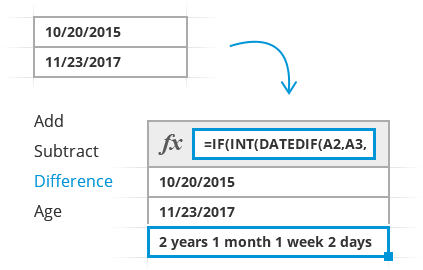## With Date & Time Formula Wizard you will

 Build formulas for dates in ExcelJust choose the operation and cells and let the add-in create a custom-tailored formula for you. Add and subtract datesAdd a desired number of years, months, weeks and days to a date, or subtract any of these units. Calculate difference between two datesChoose one of 15 combinations to calculate the difference between two dates in years, months, weeks, days or any combination of these units. Compute dates and times in one goAdd or subtract not only date values, but also hours, minutes, and seconds, all with a single formula. Convert birthday to ageUse the age calculator to get a person's age from their date of birth based on today's date or a specific date. Get reusable formulasOnce you get the formula, you can use it on any computer without the add-in installed. The tool uses only Excel math and no custom worksheet functions.

### Build formulas to calculate dates and times in Excel

Watch this short video to learn the quickest way to calculate dates in Excel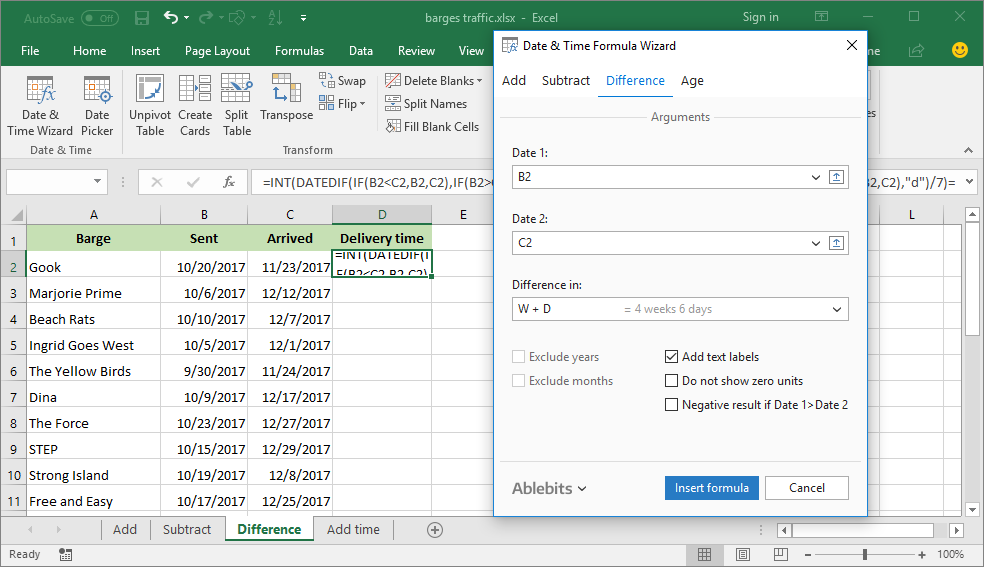Calculate the difference between two dates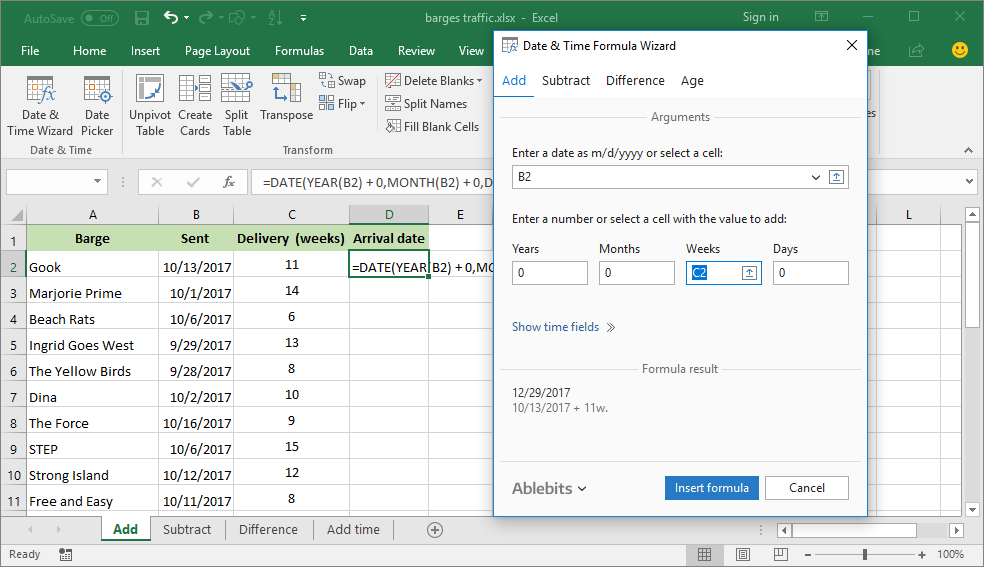Add a given number of weeks to a date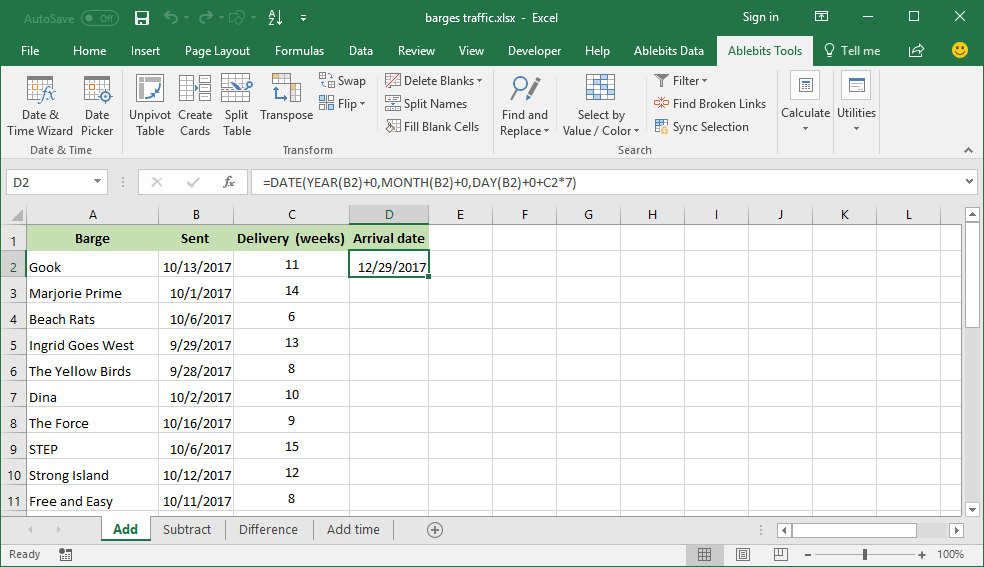A formula to add a specified number of weeks to a date is built automatically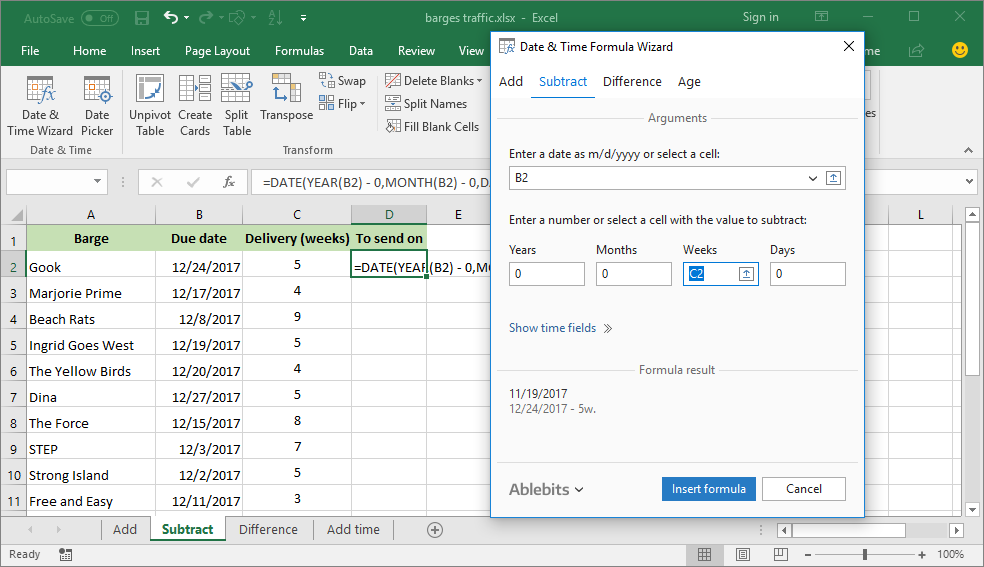Build a formula to subtract weeks from a date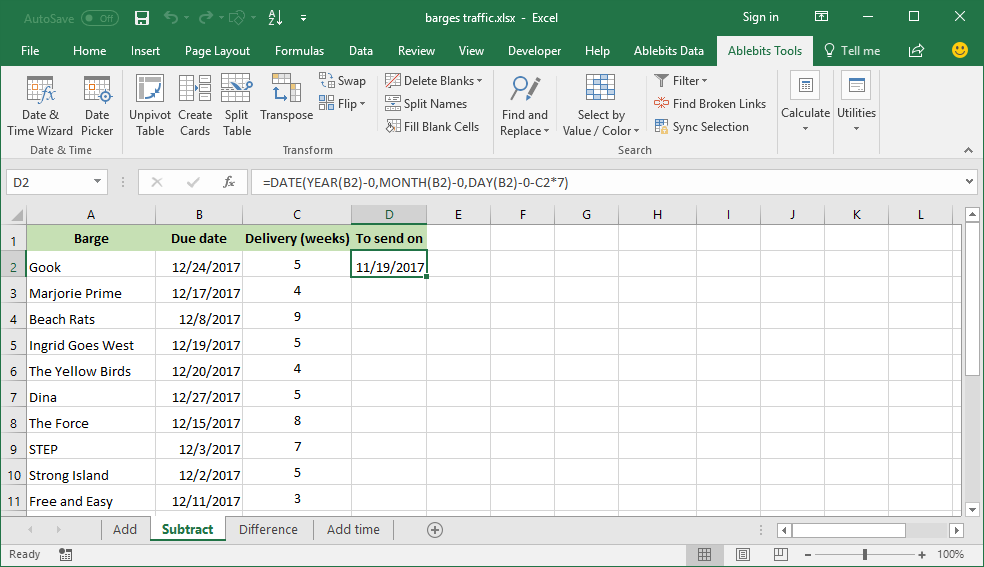A formula to subtract a specified number of weeks from a date is inserted in a selected cell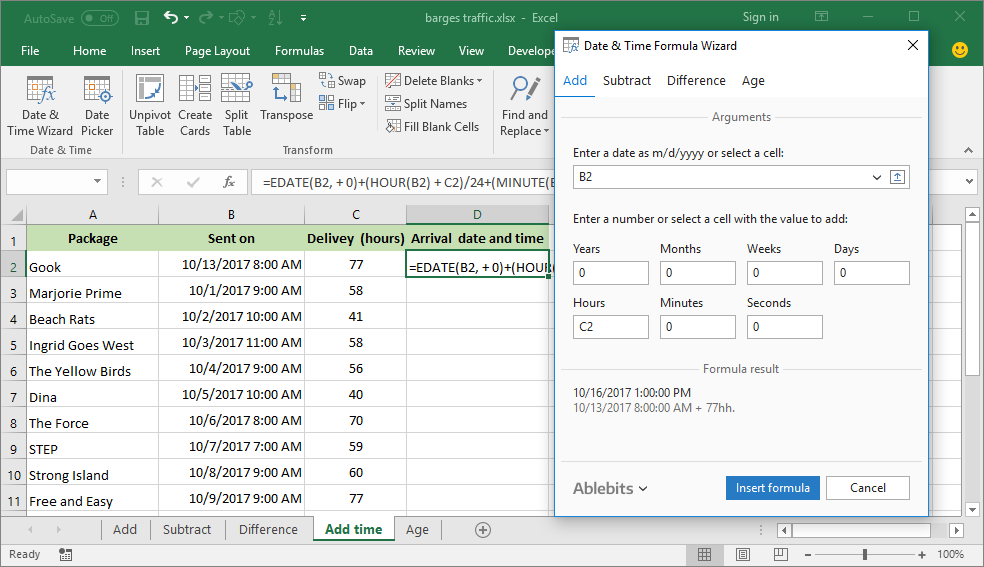Add hours to a specific date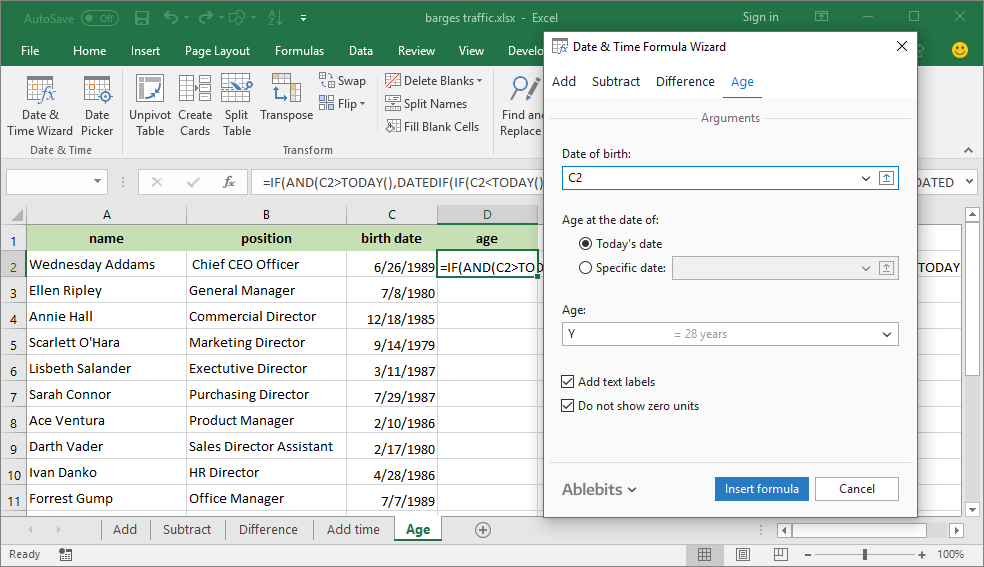Calculate age from the date of birth

## Your personal Excel date & time functions expert

### Add and subtract dates, calculate date difference and age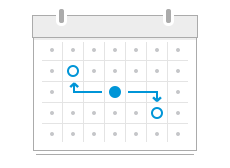Add or subtractyears, months, weeks, days orhours, minutes, seconds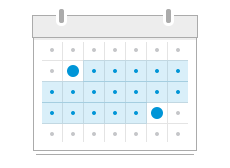Calculate the differencebetween two dates and get age from birthdateGet the results of your date & time calculations as ready-to-use formulas

### Our customers get

 Unconditional 60-day money-back guarantee Secure paymentguarantee Life-time license,no additional fees ever Volume discounts: the more you buy, the less you pay 1 license for 2 or 5 PCs or 1 server Free life-time support
 Learn more This tool is part of Ultimate Suite 60+ professional tools. No garbage. Buy now Buy as part of Ultimate Suite 60+ professional tools. No garbage.

## These companies choose Ablebits products

### About Date & Time Formula Wizard

• What is Date & Time Formula Wizard and what do I need it for?

Date & Time Formula Wizard is a formula builder for Microsoft Excel. It creates formulas to calculate the difference between two dates, add or subtract years, months, weeks, days or even hours, minutes and seconds.

• Can't I do the same without any add-ins?

Sure, you can write all the formulas yourself using Excel alone. The formula builder uses only Excel math and no custom worksheet functions. It just simplifies and accelerates this process, dramatically saving your time.

To add or subtract, first you should select the cell where you want to put the result. Then you run the add-in, enter the date or select a date cell and enter the quantity or select cells with years, months, weeks, days, hours, minutes and seconds. You will see the result of the calculation under Formula result. Click the Insert formula button. The formula will be inserted in the selected cell.

• What about the Excel date difference calculation?

If you need to calculate the difference between two dates, go to the Difference tab. There you will see three boxes to fill in: Date 1, Date 2 and Difference in. Unlike the Excel DATEDIFF function, our Date & Time Formula Wizard does not care about the order of the dates (start date and end date), it can output the result as a positive or negative number. After entering the dates, you select a format.

For instance, you can calculate the number of days between two dates; or show the difference in years, weeks and months; or weeks and days; etc. Once you click the Insert Formula button, the formula displayed in the Formula result section is inserted in the selected cell.

The detailed steps can be found here.

• How to calculate age in Excel with Date & Time Formula Wizard?

To create a formula to calculate age in Excel, switch to the Age tab. Select a cell with the birth date or enter it manually. Choose, whether you want to calculate age at today's date or a specific date. On the preview pane you will see the calculation result. Click Insert formula to get the Excel age formula inserted in your worksheet.

• In what formats can I have the date difference displayed?

The tool can calculate the difference between two dates in 15 formats:

 Years Months Weeks Days Years + months Years + weeks Years + days Months + weeks Months + days Weeks + days Years + months + weeks Years + months + days Years + weeks + days Months + weeks + days Years + months + weeks + days
• Can I get the difference between two dates with text labels?

Sure. When calculating the date difference in two or more units, select the Add text labels option to make the result more understandable.

• I want to know only the number of weeks or days between two dates, is it possible?

Yes. You can select a single unit in the Difference in box. Or, you can remove years and months from your calculations by selecting either the Exclude years or Exclude months option.

This tool is part of Ultimate Suite

## Supported versions

#### Excel

• Excel 2019
• Excel 2016
• Excel 2013
• Excel 2010
• Excel 2007
• 32-bit and 64-bit
• Desktop Excel included in Office 365
• Desktop Excel included in Office 365 ProPlus

#### Windows

• Windows 10
• Windows 8.1
• Windows 8
• Windows 7
• 32-bit and 64-bit
• Buy as part of Ultimate Suite
• 60+ professional tools. No garbage.